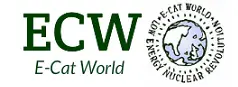# E-Cat ‘Not Fusion or Fission’ Rossi on the Misunderstood Electon

In a couple of recent comments on the Journal of Nuclear Physics, Andrea Rossi has stated that there is ‘not fusion’ in the E-Cat reaction. After these comments, Steven Karels pressed him on the topic

I understand there are two primary ways of deriving energy from the nucleus – fission and fusion. I suspect you are concerned with protecting your confidential business secrets and that is fine and understandable.

But the implication that I would draw from your comment is that the reaction is fission which cannot be the correct answer because of the low atomic number of the ingredients.

Rossi responded: :There are other nuclear interactions, besides fusion and fission.
http://www.researchgate.net/publication/330601653_E-Cat_SK_and_long_range_particle_interactions:

In another comment he wrote:

We are still in the Middle Age of Physics, because the Standard Model did not understand what is an electron, while Albert Einstein said ” we will understand Physics when we will understand electrons”.

Also this:

Einstein wrote: ”to understand electron means to understand Physicst“.
This is the issue I am focused on because from the study of it depends failure or success of the attempt I am making to get electricity directly from the plasma.

He has cited this book as being of interest to him: “Maxwell-Dirac Theory and Occam’s Razor: Unified Field, Elementary Particles, and Nuclear Interactions ” by Alexander Unziker” https://www.amazon.com/Maxwell-Dirac-Theory-Occams-Razor-Interactions/dp/9529420927/

Below are 17 points that Andrea Rossi sent me summarizing the contents of the book:

Here below some of the key points presented in the book:

1) Does exist a a scalar field that is the four divergence of the four electromagnetic potential
2) Charge density is the partial time derivative of the scalar field
3) Current density vector components are the partial space derivatives of the scalar field
4) D’Alembert equation can be applied to both scalar field and charge density
5) Electron mass is the angular frequency of a rotating elementary charge along a circumference which length is equal to Compton wavelength
6) Electron charge follows an elicoidal motion that can be encoded with a single spinor of Cl(3,1).
7)The concept of relativistic mass is easily derived from the electron charge elicoidal motion.
8) The electron charge is distributed on the surface of a sphere that follows a circular or elicoidal motion.
9)The value of the elementary charge is equal (in natural units) to the square root of the radius of this sphere with the radius of the charge orbit
10) The electron spin 1/2 is the quantized component along an external magnetic field of electron angular momentum (=hbar)
11) The elementary charge e is always associate to a quantized magnetic flux phi=h/e
12) The electron charge has a pure electromagnetic momentum eA equal to its mass in natural units
13) Newton law F=ma can be easily explained inside the theory framework
14) The charge moves at speed of light
15) Heisenberg uncertainty principle has a simple geometric interpretation
16) Ultradense hydrogen (and consequently AHE and LENR experiments) may be explained with the formations of pseudo-neutrons where the proton is at center of electron Zitterbewegung charge orbit
17) The electron model suggests a pure electromagnetic-geometric interpretationof Proca, Klein-Gordon, Dirac and Aharonov-Bohm equations